Function Repository Resource:

# PerfectPower

Find all perfect power representations of an integer

Contributed by: Swastik Banerjee
 ResourceFunction["PerfectPower"][n] gives a list of all nontrivial integer base and exponent pairs that equal n.

## Details and Options

An integer base and exponent represent another integer nontrivially if both base and exponent are greater than one.
An integer has a perfect power if the set of exponents in its prime factorization have a GCD greater than one.
ResourceFunction["PerfectPower"] returns a List of all pairs {xi,ki} with xi>1 and ki>1 such that n=xik1. If there are no such representations, it returns an empty List.

## Examples

### Basic Examples (1)

Integer base and exponent pairs that can be used to represent 16 as a perfect power:

 In:=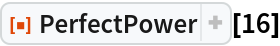Out=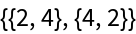### Scope (2)

Find all numbers less than or equal to 105 that can be represented as perfect powers of exactly three numbers:

 In:=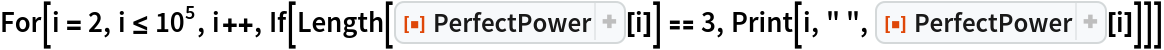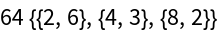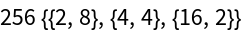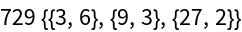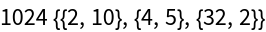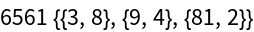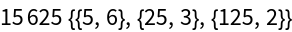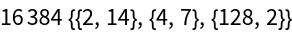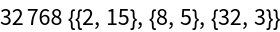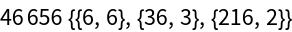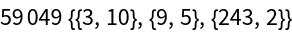Yet another example with a large number:

 In:=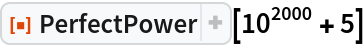Out=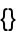### Applications (1)

Many of Pillai's conjectures or Catalan's proof can be investigated and verified using PerfectPower. Investigating solutions for the Catalan equation:

 In:=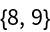### Properties and Relations (1)

PerfectPower of primes always returns an empty List:

 In:=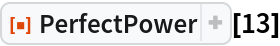Out=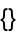### Possible Issues (1)

PerfectPower[n] returns unevaluated n is negative:

 In:=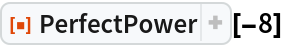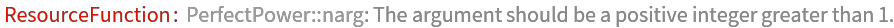Out=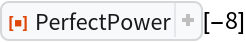### Neat Examples (2)

1267650600228229401496703205376 decomposed into perfect powers of integers:

 In:=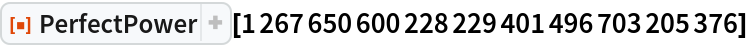Out=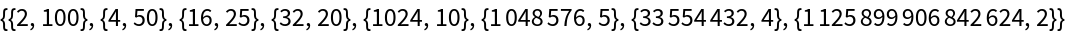PerfectPower representations of a fairly large number:

 In:=Out=## Publisher

Wolfram Summer School

## Version History

• 1.0.0 – 25 September 2020